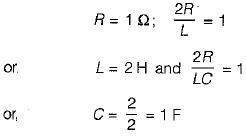Courses

# Test: Second Order RLC Circuits

## 10 Questions MCQ Test Topicwise Question Bank for Electrical Engineering | Test: Second Order RLC Circuits

Description
This mock test of Test: Second Order RLC Circuits for Electrical Engineering (EE) helps you for every Electrical Engineering (EE) entrance exam. This contains 10 Multiple Choice Questions for Electrical Engineering (EE) Test: Second Order RLC Circuits (mcq) to study with solutions a complete question bank. The solved questions answers in this Test: Second Order RLC Circuits quiz give you a good mix of easy questions and tough questions. Electrical Engineering (EE) students definitely take this Test: Second Order RLC Circuits exercise for a better result in the exam. You can find other Test: Second Order RLC Circuits extra questions, long questions & short questions for Electrical Engineering (EE) on EduRev as well by searching above.
QUESTION: 1

### A current is given by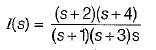The initial and final values of currents are respectively

Solution: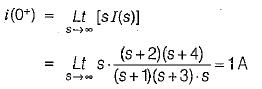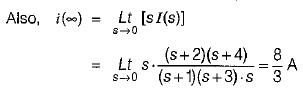QUESTION: 2

Solution:
QUESTION: 3

### For the parallel RLC circuit to be underdamped

Solution:

Characteristic equation of a parallel RLC circuit is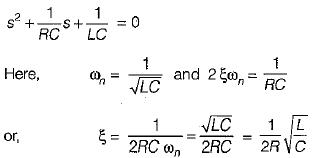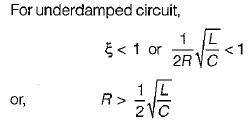QUESTION: 4

For the circuit shown below, the switch K is closed at t = 0,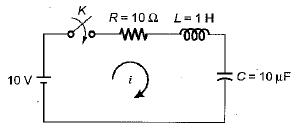The value of the current and their derivatives for the above circuit at t = 0 were foundout and matched as given below: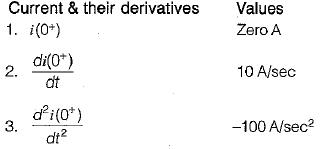Which of the above are correctly matched ?

Solution:

At t = 0+, inductor will act as open circuit therefore, i(0+) = 0 A.
Applying KVL in the loop, we have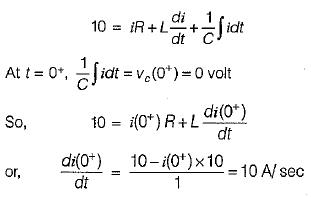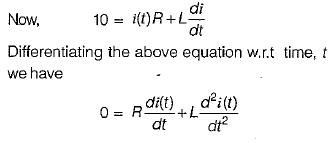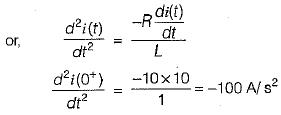QUESTION: 5

The energy in a network in Laplace domain is given by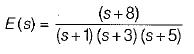​The initial and final values of power are respectively

Solution:

We know that,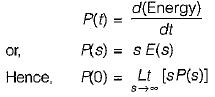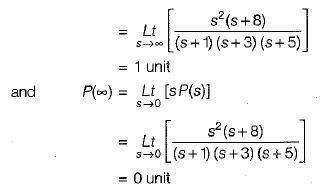QUESTION: 6

A voltage is given by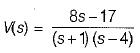The value of V(t→ ∞) is

Solution: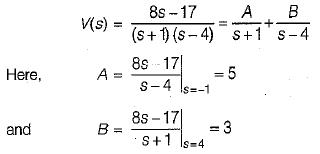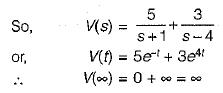QUESTION: 7

The voltage across a capacitor is given by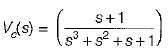If the capacitor has the value of 2 F, initial value of current through it (at t = 0+) will be

Solution: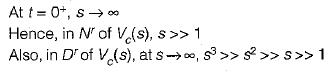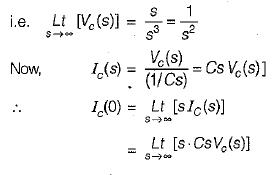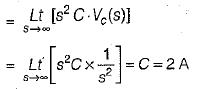Hence, initial value of current through the capacitor = 2 A.

QUESTION: 8

The impedance Z(s) in the circuit shown below is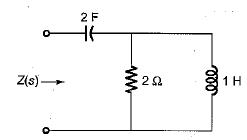Solution:

The given circuit in Laplace domain is shown below.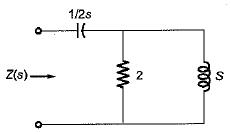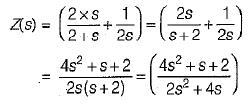QUESTION: 9

The voltage Vc1,Vc2 and Vc3 across the capacitors in the circuit shown below under steady-state, are respectively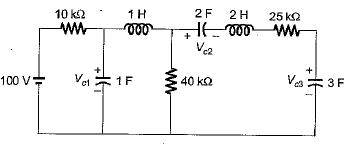Solution:

Under steady state condition inductors will act as short circuit while capacitors will act as open circuit.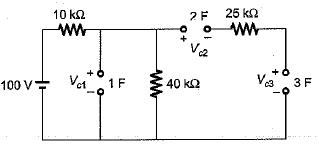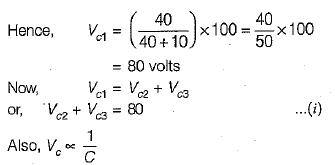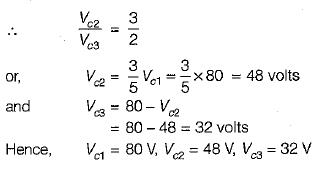QUESTION: 10

The value of Z(s) in the circuit shown below is given by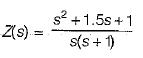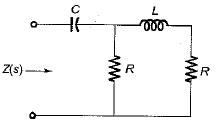The values of R, L and Care respectively given by

Solution: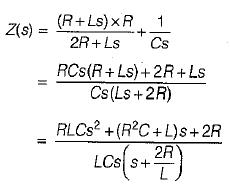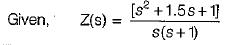On comparing the two values of Z(s), we get